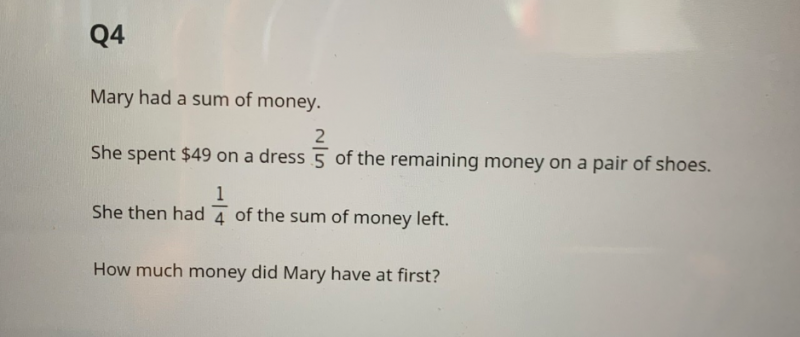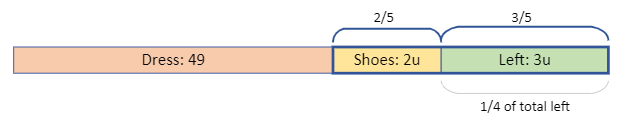# Question```The remaining money after spending \$49 on dress is the box with blue border.
As 2/5 of it is spent on shoes, we can write it as 2u vs 3u.

Working:
Dress = 49
Shoes = 2u  (2/5 of remaining)
Left  = 3u  (is also 1/4 of total)
Amount left = 3u = 1/4 of total. So total is 4 x 3u = 12u.
Thus Dress is 12u - 5u = 7u = 49 and u = 7.
So Mary had 12u = 12 x 7 = \$84 at first.

Check:
Remaining after dress: 84 - 49 = 35
Shoes = 2 x 7 = 14 and 14/35 = 2/5.
Left = 35 - 14 = 21 and 21/84 = 1/4.```

Thank you.

Let u be the total money at first.

[       Dress    ][            Shoes            ][     Left      ]

[ ——49——-][——(2/5)(u-49)——–][—–1/4u—]

[——————-(3/4)u———————]

49 + (2/5)(u-49) = (3/4)u     ( x 20 on both sides)

980 +8(u-49) = 15u

7u = 980-392 = 588

u = 588/7 = 84

Hence Mary has \$84 at first.

0 Replies 1 Like

The key point to solve this problem is to be very clear of 2/5 and ¼ are referring to different amount of money.

2/5 is referring to remaining money which is ( sum of money-49 ) and ¼ is referring to the sum of money.

Let the sum of Money be S.

S – 2/5*(S-49) – 49= ¼*S

S*(1-2/5-1/4)=49*3/5

7/20*S=49*3/5

S=84

So the sum of money is \$84.

0 Replies 1 Like